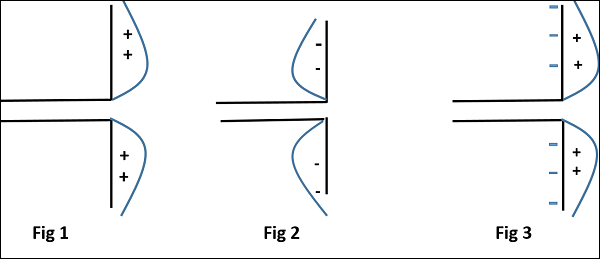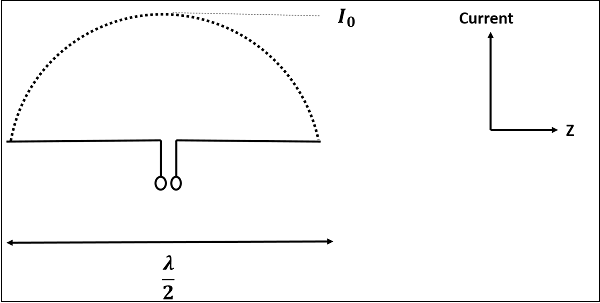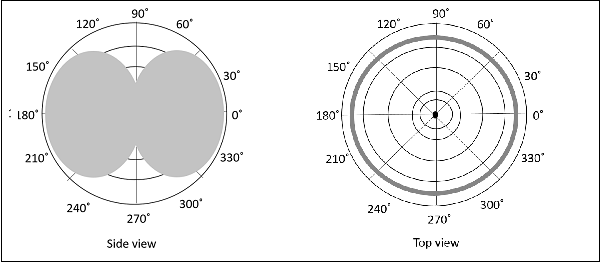# Antenna Theory - Half-Wave Dipole

The dipole antenna is cut and bent for effective radiation. The length of the total wire, which is being used as a dipole, equals half of the wavelength (i.e., l = λ/2). Such an antenna is called as half-wave dipole antenna. This is the most widely used antenna because of its advantages. It is also known as Hertz antenna.

### Frequency range

The range of frequency in which half-wave dipole operates is around 3KHz to 300GHz. This is mostly used in radio receivers.

## Construction & Working of Half-wave Dipole

It is a normal dipole antenna, where the frequency of its operation is half of its wavelength. Hence, it is called as half-wave dipole antenna.

The edge of the dipole has maximum voltage. This voltage is alternating (AC) in nature. At the positive peak of the voltage, the electrons tend to move in one direction and at the negative peak, the electrons move in the other direction. This can be explained by the figures given below.The figures given above show the working of a half-wave dipole.

• Fig 1 shows the dipole when the charges induced are in positive half cycle. Now the electrons tend to move towards the charge.

• Fig 2 shows the dipole with negative charges induced. The electrons here tend to move away from the dipole.

• Fig 3 shows the dipole with next positive half cycle. Hence, the electrons again move towards the charge.

The cumulative effect of this produces a varying field effect which gets radiated in the same pattern produced on it. Hence, the output would be an effective radiation following the cycles of the output voltage pattern. Thus, a half-wave dipole radiates effectively.The above figure shows the current distribution in half wave dipole. The directivity of half wave dipole is 2.15dBi, which is reasonably good. Where, ‘i’ represents the isotropic radiation.

The radiation pattern of this half-wave dipole is Omni-directional in the H-plane. It is desirable for many applications such as mobile communications, radio receivers etc.The above figure indicates the radiation pattern of a half wave dipole in both H-plane and V-plane.

The radius of the dipole does not affect its input impedance in this half wave dipole, because the length of this dipole is half wave and it is the first resonant length. An antenna works effectively at its resonant frequency, which occurs at its resonant length.

The following are the advantages of half-wave dipole antenna −

• Input impedance is not sensitive.

• Matches well with transmission line impedance.

• Has reasonable length.

• Length of the antenna matches with size and directivity.

The following are the disadvantages of half-wave dipole antenna −

• Not much effective due to single element.

• It can work better only with a combination.

### Applications

The following are the applications of half-wave dipole antenna −